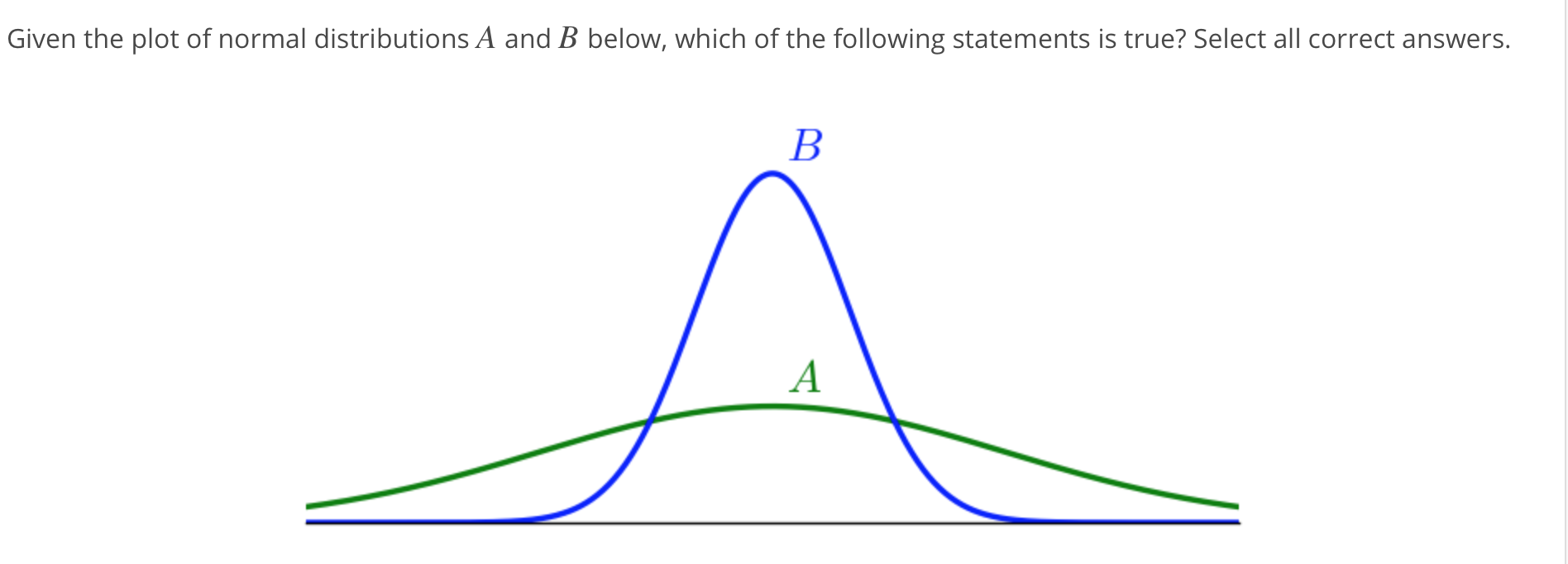# Given the plot of normal distributions A and B below, which of the following statements is true? Select all correct answers

Question
Given the plot of normal distributions A and B below, which of the following statements is true? Select all correct answers.

A normal bell curve labeled Upper A and a normal elongated curve labeled Upper B are centered at the same point. Normal curve Upper B is narrower and above normal curve Upper A.

Select all that apply:

•
A has the larger mean.
•
•
B has the larger mean.
•
•
The means of A and B are equal.
•
•
A has the larger standard deviation.
•
•
B has the larger standard deviation.
•
•
The standard deviations of A and B are equal.help_outlineImage TranscriptioncloseGiven the plot of normal distributions A and B below, which of the following statements is true? Select all correct answers fullscreen
check_circle

We are given that the both the normal curves A and B are centered at the same point. Therefore, both the curves have same mean. Also, we are given that the normal curve B is narrower than the normal curve A. Therefore, the normal curve A has larger standard deviation.

Therefore, the correct options are:

The means of A and B are equal.

A...

### Want to see the full answer?

See Solution

#### Want to see this answer and more?

Solutions are written by subject experts who are available 24/7. Questions are typically answered within 1 hour.*

See Solution
*Response times may vary by subject and question.
Tagged in

### Other